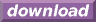#Calcute: freeware calculator

## Hyperbolic Secant (sech)

Calcute implements the hyperbolic secant function with the sech function.

```sech(1)
0.648054273664
```

It is defined as the inverse of the hyperbolic cosine function sech(x) = 1 / cosh(x) or equivalently sech(x) = 2) / (exp(x) + exp(-x)). Note that this definition refers to a multiplicative inverse; the hyperbolic secant is not the same as the inverse hyperbolic cosine (acosh) function.